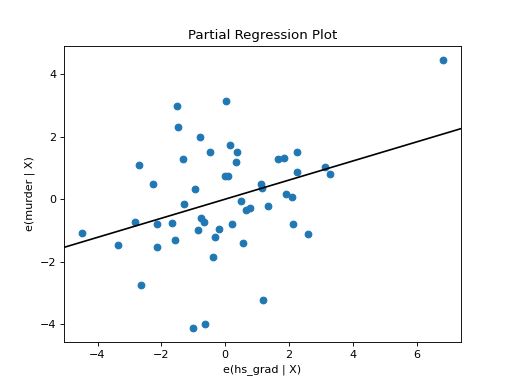# statsmodels.graphics.regressionplots.plot_partregress¶

statsmodels.graphics.regressionplots.plot_partregress(endog, exog_i, exog_others, data=None, title_kwargs={}, obs_labels=True, label_kwargs={}, ax=None, ret_coords=False, **kwargs)[source]

Plot partial regression for a single regressor.

Parameters
endog

The endogenous or response variable. If string is given, you can use a arbitrary translations as with a formula.

exog_i

The exogenous, explanatory variable. If string is given, you can use a arbitrary translations as with a formula.

exog_others

Any other exogenous, explanatory variables. If a list of strings is given, each item is a term in formula. You can use a arbitrary translations as with a formula. The effect of these variables will be removed by OLS regression.

data

Some kind of data structure with names if the other variables are given as strings.

title_kwargsdict

Keyword arguments to pass on for the title. The key to control the fonts is fontdict.

obs_labels

Whether or not to annotate the plot points with their observation labels. If obs_labels is a boolean, the point labels will try to do the right thing. First it will try to use the index of data, then fall back to the index of exog_i. Alternatively, you may give an array-like object corresponding to the observation numbers.

label_kwargsdict

Keyword arguments that control annotate for the observation labels.

axAxesSubplot, optional

If given, this subplot is used to plot in instead of a new figure being created.

ret_coordsbool

If True will return the coordinates of the points in the plot. You can use this to add your own annotations.

**kwargs

The keyword arguments passed to plot for the points.

Returns
figFigure

If ax is None, the created figure. Otherwise the figure to which ax is connected.

coordslist, optional

If ret_coords is True, return a tuple of arrays (x_coords, y_coords).

plot_partregress_grid

Plot partial regression for a set of regressors.

Notes

The slope of the fitted line is the that of exog_i in the full multiple regression. The individual points can be used to assess the influence of points on the estimated coefficient.

Examples

Load the Statewide Crime data set and plot partial regression of the rate of high school graduation (hs_grad) on the murder rate(murder).

The effects of the percent of the population living in urban areas (urban), below the poverty line (poverty) , and in a single person household (single) are removed by OLS regression.

>>> import statsmodels.api as sm
>>> import matplotlib.pyplot as plt

>>> crime_data = sm.datasets.statecrime.load_pandas()